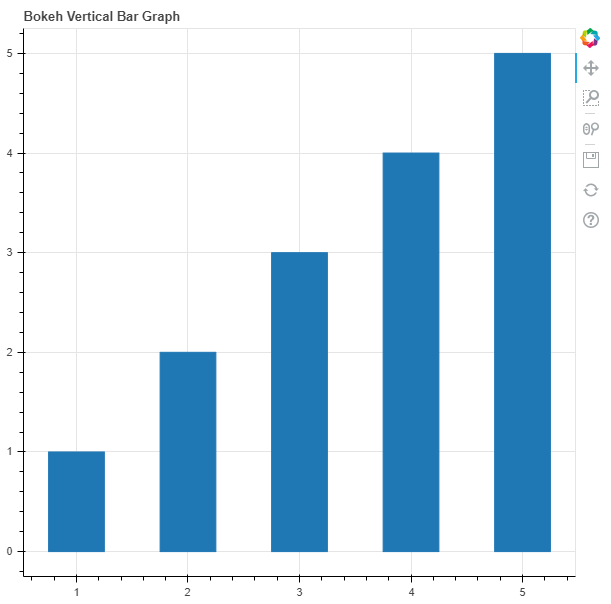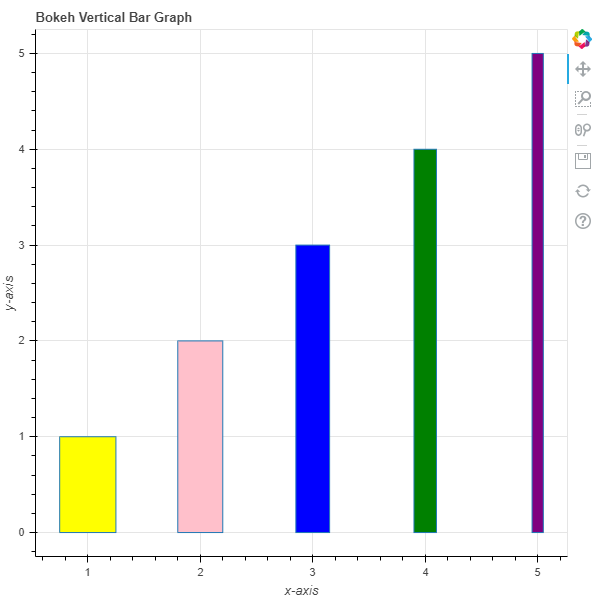# Python Bokeh – Plotting Vertical Bar Graphs

• Difficulty Level : Easy
• Last Updated : 30 Nov, 2021

Bokeh is a Python interactive data visualization. It renders its plots using HTML and JavaScript. It targets modern web browsers for presentation providing elegant, concise construction of novel graphics with high-performance interactivity.
Bokeh can be used to plot vertical bar graphs. Plotting vertical bar graphs can be done using the vbar() method of the plotting module.

## plotting.figure.vbar()

Syntax : vbar(parameters)
Parameters :

• x : x-coordinates of the center of the vertical bars
• width : thickness of the vertical bars
• top : y-coordinates of the top edges
• bottom : y-coordinates of the bottom edges, default is 0
• fill_alpha : fill alpha value of the vertical bars
• fill_color : fill color value of the vertical bars
• hatch_alpha : hatch alpha value of the vertical bars, default is 1
• hatch_color : hatch color value of the vertical bars, default is black
• hatch_extra : hatch extra value of the vertical bars
• hatch_pattern : hatch pattern value of the vertical bars
• hatch_scale : hatch scale value of the vertical bars, default is 12
• hatch_weight : hatch weight value of the vertical bars, default is 1
• line_alpha : percentage value of line alpha, default is 1
• line_cap : value of line cap for the line, default is butt
• line_color : color of the line, default is black
• line_dash : value of line dash such as :
• solid
• dashed
• dotted
• dotdash
• dashdot

default is solid

• line_dash_offset : value of line dash offset, default is 0
• line_join : value of line join, default in bevel
• line_width : value of the width of the line, default is 1
• name : user-supplied name for the model
• tags : user-supplied values for the model

Other Parameters :

• alpha : sets all alpha keyword arguments at once
• color : sets all color keyword arguments at once
• legend_field : name of a column in the data source that should be used
• legend_group : name of a column in the data source that should be used
• legend_label : labels the legend entry
• muted : determines whether the glyph should be rendered as muted or not, default is False
• name : optional user-supplied name to attach to the renderer
• source : user-supplied data source
• view : view for filtering the data source
• visible : determines whether the glyph should be rendered or not, default is True
• x_range_name : name of an extra range to use for mapping x-coordinates
• y_range_name : name of an extra range to use for mapping y-coordinates
• level : specifies the render level order for this glyph

Returns : an object of class GlyphRenderer

Example 1 :In this example we will be using the default values for plotting the graph.

## Python3

 `# importing the modules``from` `bokeh.plotting ``import` `figure, output_file, show`` ` `# file to save the model``output_file(``"gfg.html"``)``     ` `# instantiating the figure object``graph ``=` `figure(title ``=` `"Bokeh Vertical Bar Graph"``)`` ` `# x-coordinates to be plotted``x ``=` `[``1``, ``2``, ``3``, ``4``, ``5``]`` ` `# x-coordinates of the top edges``top ``=` `[``1``, ``2``, ``3``, ``4``, ``5``]`` ` `# width / thickness of the bars``width ``=` `0.5`` ` `# plotting the graph``graph.vbar(x,``           ``top ``=` `top,``           ``width ``=` `width)`` ` `# displaying the model``show(graph)`

Output :Example 2 :In this example we will be plotting vertical bars with different parameters.

## Python3

 `# importing the modules``from` `bokeh.plotting ``import` `figure, output_file, show`` ` `# file to save the model``output_file(``"gfg.html"``)``     ` `# instantiating the figure object``graph ``=` `figure(title ``=` `"Bokeh Vertical Bar Graph"``)` `# name of the x-axis``graph.xaxis.axis_label ``=` `"x-axis"``     ` `# name of the y-axis``graph.yaxis.axis_label ``=` `"y-axis"`` ` `# x-coordinates to be plotted``x ``=` `[``1``, ``2``, ``3``, ``4``, ``5``]`` ` `# x-coordinates of the top edges``top ``=` `[``1``, ``2``, ``3``, ``4``, ``5``]`` ` `# width / thickness of the bars``width ``=` `[``0.5``, ``0.4``, ``0.3``, ``0.2``, ``0.1``]` `# color values of the bars``fill_color ``=` `[``"yellow"``, ``"pink"``, ``"blue"``, ``"green"``, ``"purple"``]`` ` `# plotting the graph``graph.vbar(x,``           ``top ``=` `top,``           ``width ``=` `width,``           ``fill_color ``=` `fill_color)`` ` `# displaying the model``show(graph)`

Output :My Personal Notes arrow_drop_up